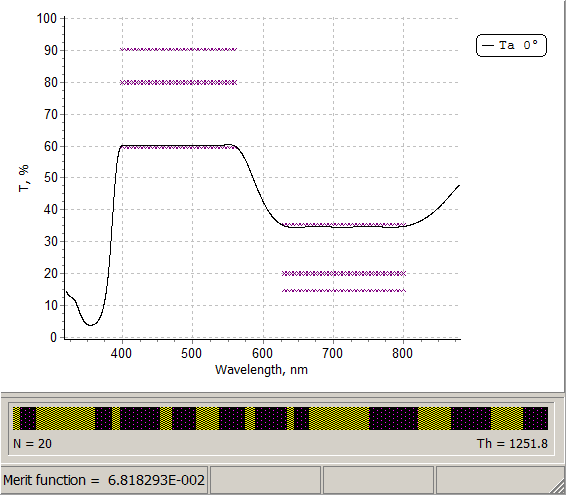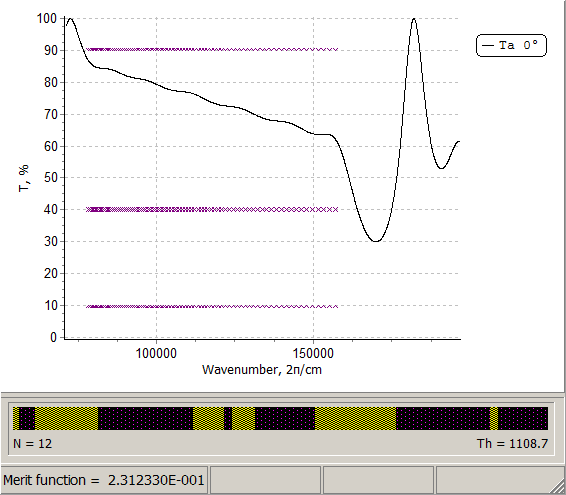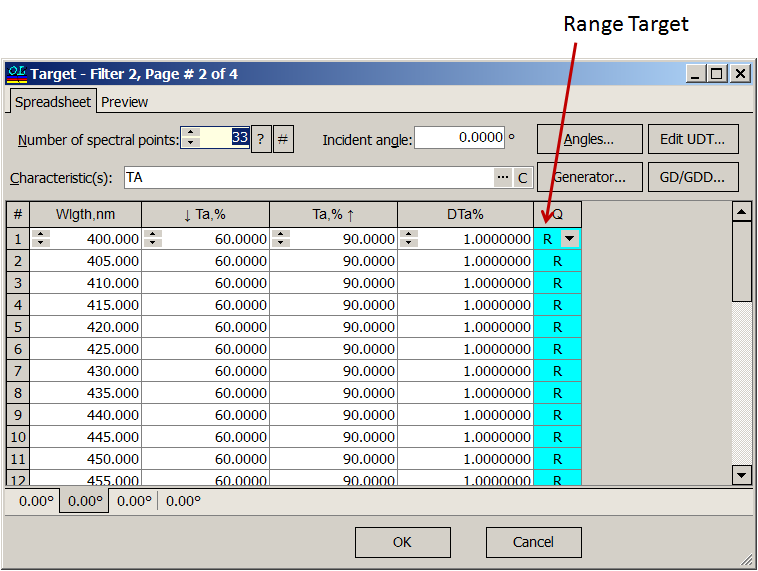### Floating Constants

 A new big addition to OptiLayer design capabilities: supporting floating constants for all targets. It is possible to specify only constant offset, or more complicated offsets depending on frequency: $$C_1+C_2\cdot\omega$$ or $$C_1+C_2\cdot\omega+C_3\cdot\omega^2$$. These new options are important for designing of ultrafast coatings in the case when a constant/linear/parabolic GD/GDD are required. The option is useful also in general case, when, for example, it is necessary to design a coating with constant T/R in some spectral region, and the absolute value of this characteristic is not important. Example (right panel): target transmittance is to be flat in the spectral range in the visible spectral range; transmittance must be in the corridor from 60% to 90% and from 15% to 35% in the spectral ranges from 400 to 570 nm and from 630 to 800 nm, respectively.There are three types of floating constants: $$C_1$$ - spectral characteristics are as flat as possible; $$C_1+C_2\cdot\omega$$ - spectral characteristics are linear with respect to the frequency $$\omega$$ (example in the left panel);  $$C_1+C_2\cdot\omega+C_3\cdot\omega^2$$   - spectral characteristics are parabolic with respect to $$\omega$$ Target specification: you should specify two pages for each desired spectral range. In the first page you should indicate a floating constant type: In the second page you specify the allowed limits of the spectral characteristics using range targets: